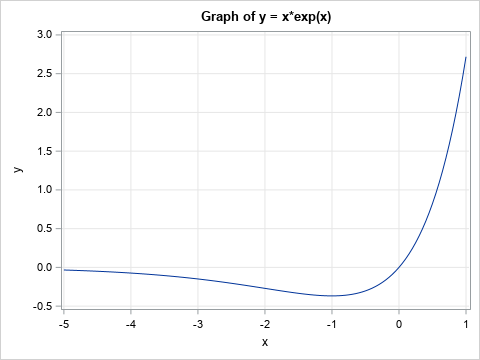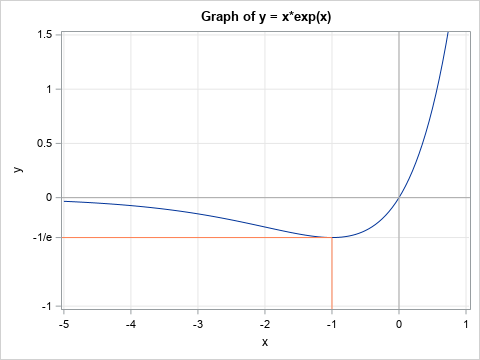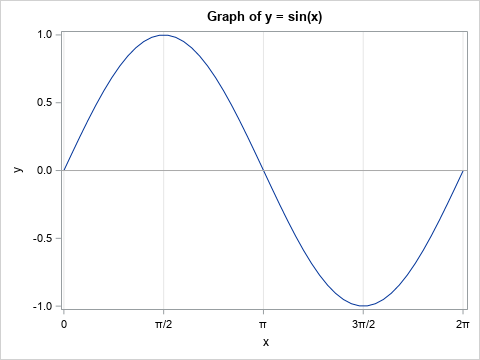When you create a graph by using the SGPLOT procedure in SAS, usually the default tick locations are acceptable. Sometimes, however, you might want to specify a set of custom tick values for one or both axes. This article shows three examples:

• Specify evenly spaced values.
• Specify tick values that are not evenly spaced.
• Specify custom labels for the ticks, including symbols such as π.

You can accomplish these tasks by using options on the XAXIS and YAXIS statements. The VALUES= option enables you to specify tick locations; the VALUESDISPLAY= option enables you to specify text strings to display on an axis.

### Use the VALUES= option to specify tick locations

You can use the VALUES= option on the XAXIS or YAXIS statement to specify a set of values at which to display ticks. If you want to specify a set of evenly spaced values, you can use the (start TO stop BY increment) syntax. For example, the following call to PROC SGPLOT specifies that the ticks on the Y axis should be specified at the locations -0.5, 0, 0.5, 1, ..., 3.

```/* create data for the graph of the function y = x*exp(x) */ data Function; do x = -5 to 1 by 0.05; y = x*exp(x); output; end; run;   ods graphics / width=480px height=360px; title "Graph of y = x*exp(x)"; proc sgplot data=Function; series x=x y=y; yaxis grid values=(-0.5 to 3 by 0.5); /* specify tick locations */ xaxis grid; run;```Notice that the Y axis has tick marks at the values that are specified by the VALUES= option.

### Specify tick mark locations and labels

Sometimes you might want to highlight a specific value on a graph. For example, you might want to indicate where a graph has a maximum, minimum, or inflection point. The graph in the previous section has a minimum value at (x,y) = (1, -1/e) ≈ (1, -0.368). You can use the VALUES= option to specify a set of tick locations that include -0.368. Because nobody who reads the graph will associate -0.368 with the number -1/e, you might want to display the text string "-1/e" on the axis instead of -0.368.

The VALUESDISPLAY= option enables you to specify a text string for each value in the VALUES= list. The strings are used as labels for each tick mark. For example, the following call to PROC SGPLOT uses the VALUESDISPLAY= option to display the string "-1/e" at the location y = -0.368. To further emphasize that the value corresponds to the minimum value of the function, I use the DROPLINE statement to display line segments that extend from the point (1, -0,368) to each axis.

```%let eInv = %sysfunc(exp(-1)); /* e inverse = exp(-1) */ title "Graph of y = x*exp(x)"; proc sgplot data=Function; series x=x y=y; dropline x= -1 y= -&eInv / dropto=both lineattrs=(color=coral); /* Draw coordinate axes in dark grey */ refline 0 / axis=y lineattrs=(color=darkgrey); refline 0 / axis=x lineattrs=(color=darkgrey); /* ticks on the Y axis include -1/e */ yaxis grid max=1.5 values = (-1 -&eInv 0 0.5 1 1.5 ) /* locations */ valuesdisplay = ("-1" "-1/e" "0" "0.5" "1" "1.5"); /* strings to display */ xaxis grid; run;```### Use symbols for tick labels

The previous example displays the string "-1/e" at a tick mark. You can also display symbols on the axes. Back in 2011, Dan Heath discussed how to specify symbols by using Unicode values. Since SAS 9.4M3, you can also use Unicode symbols in user-defined formats. The following example defines the Unicode symbol for pi (π) and uses the symbol in the VALUESDISPLAY= option to label tick marks at π/2, π, 3π/2, and 2π.

```/* create the graph of y = sin(x) on [0, 2*pi] */ data Trig; pi = constant('pi'); drop pi; do x = 0 to 2*pi by pi/25; y = sin(x); output; end; run;   %let piChar = (*ESC*){Unicode pi}; /* define pi symbol */ title "Graph of y = sin(x)"; proc sgplot data=Trig noautolegend; series x=x y=y; refline 0 / axis=y lineattrs=(color=darkgrey); xaxis grid values = (0 1.57 3.14 4.71 6.28 ) /* 0, pi/2, pi, 3pi/2, 2pi */ valuesdisplay = ("0" "&piChar/2" "&piChar" "3&piChar/2" "2&piChar"); run;```In this example, I used the VALUES= and VALUESDISPLAY= option on the XAXIS statement. Consequently, the X axis displays the symbols π/2, π, 3π/2, and 2π.

In summary, you can use the VALUES= option to specify a list of locations for ticks on an axis. You can use the (start TO stop BY increment) notation to specify evenly spaced ticks. For unevenly spaced ticks, you can list the ticks manually, such as (1 5 10 25 50 100). If you want to display text instead of numbers, you can use the VALUESDISPLAY= option, which enables you to specify arbitrary strings. You can use Unicode symbols in the VALUESDISPLAY= list.

The post Add custom tick marks to a SAS graph appeared first on The DO Loop.